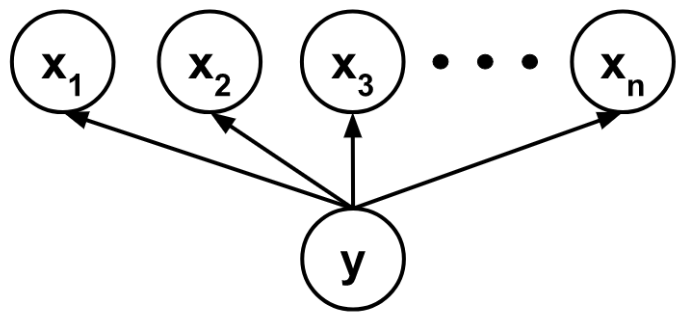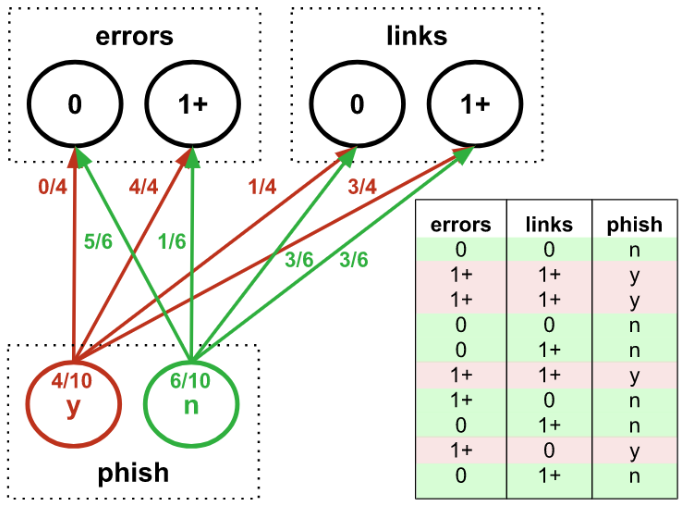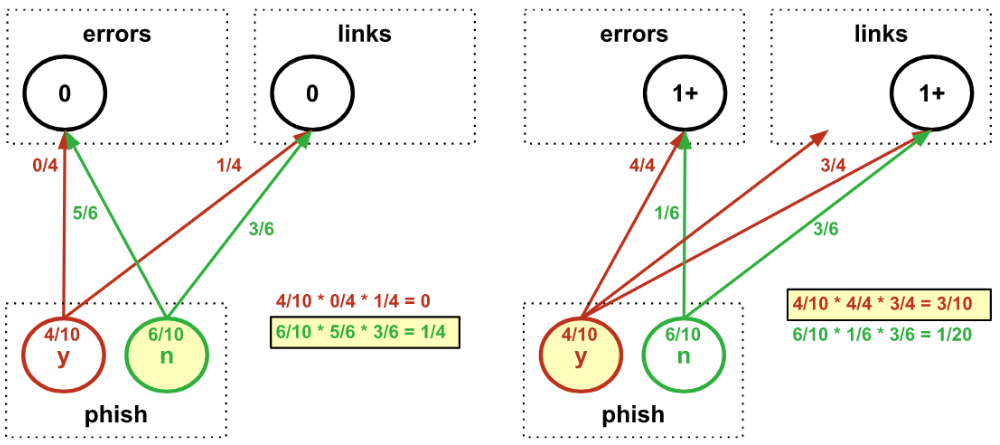# Intuiting Naive Bayes

This post is part of a series.

If we know the causal structure between variables in our data, we can build a Bayesian network, which encodes conditional dependencies between variables via a directed acyclic graph. Such a model is constrained by our human understanding of the relationship between parts of the data, though, and may not be optimal when we wish to predict a target variable despite knowing little about the other variables to which it may or may not relate.

That being said, if we know that the target variable is a class that somehow encapsulates the other variables, it can be worthwhile to try a Bayesian network where the other variables are assumed to depend conditionally and independently on the class. This is called Naive Bayes classification because it naively assumes that the presence of a particular feature in a class is unrelated to the presence of any other feature.If the data is given by $\lbrace ((x_{ij}), y_i) \rbrace$ and each $y_i$ belongs to a class $C_k,$ then the Naive Bayes classifier computes

\begin{align*} C_k &= \arg\max_{C_k} P(C_k|x) \\[5pt] &= \arg\max_{C_k}\frac{P(x|C_k)P(C_k)}{P(x)} \\[5pt] &= \arg\max_{C_k} P(x|C_k)P(C_k) \\[5pt] &= \arg\max_{C_k} P(C_k) \prod_j P(x_j|C_k) \end{align*}

For example, we could build a Naive Bayes classifier to predict whether an email is a phishing attempt based on whether it has spelling errors and links:We could then use our model to test whether a new email is a phishing attempt:In this example, we used discrete bins for the features – but Naive Bayes can also handle features that are fit to continuous distributions. And despite assuming that features are independent (and thus potentially ignoring a lot of useful information), Naive Bayes can sometimes perform well enough in simple applications to get the job done.

This post is part of a series.

Tags: